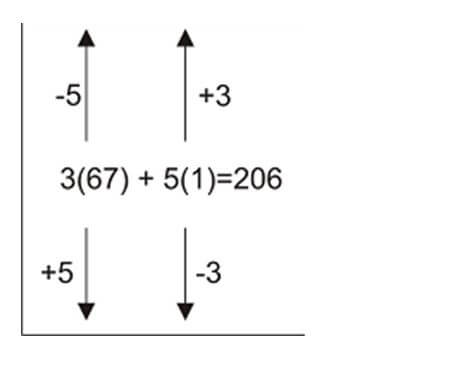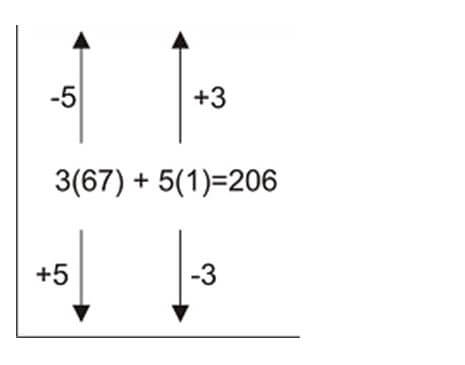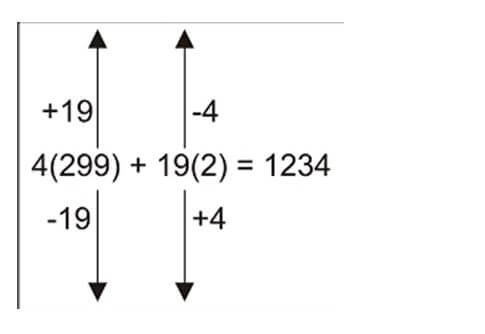This article is written by a 100-percentiler in CAT 2016. He is a director of a leading test-prep institute and is the best person to learn Mathematics from. In fact, he was the trainer for Wordpandit himself and Wordpandit credits a lot of his learning to this Master Trainer. Dig into this amazing article by this Mathematics Wizard. We will reveal his identity in the coming posts..:)

A very common question type in CAT is to find the number of integral solutions for a given equation. In fact, the title of this post is a little misleading. This question type can possibly belong to three topics:

• Number System
• P & C
• Or Algebra

Well, we need to a figure out a way to solve these problems and not necessarily the topic they belong to. So, let’s dig into this topic and figure out how to approach this question type.

How to find integral solutions for linear equation ax + by = c, where a, b and c are constants and x, y are variables whose integral values are sought?

1. Find number of positive integral solutions to the equation 3x + 5y = 206.
2. Find the number of positive integral solutions to the equation 3x – 5y = 206.
3. Find the number of positive integral solutions to the following set of equations

x + 2y + 4z = 205             and

x + 3y + 6z = 300

Let’s start with a sample question in order to explain the method of solving these types of questions.

Question 1: Find number of positive integral solutions to the equation 3x + 5y = 206.

Step 1: Always ensure that in our general equation ax + by = c, a & b are coprime.

In our sample question, the equation is 3x + 5y = 206, for which 3 and 5 are already coprime.

Had there been another equation like 2x + 8y = 200, we’d have reduced it to x + 4y = 100 by dividing the previous equation by 2, the Highest Common Factor of 2 & 8.

After this reduction of equation to the form where a & b are coprime, if a,b and c are all integers, the equation will have an infinite number of integral solutions (as you’ll see in a while). So the equations 3x + 5y = 206 and x + 4y = 100 will have infinite number of integral solutions.

On the contrary, look at the case 6x + 9y = 1000. Here 6 and 9 are not coprime and their Highest Common Factor is 3. We divide the whole equation by 3 to make them coprime. After division by 3, the equation becomes 2x + 3y = 1000/3, which clearly cannot have any integral solution at all because if x and y are integers, so should be 2x + 3y, which is not the case. This last case shows the importance of reducing the equation ax + by = c to the form where a & b are coprime.

Step 2: Find any one integral solution to the equation in concern.

Having known that there are infinite numbers of integral solutions to our equation, we’ll try to find one sample integral solution.

For our sample equation 3x + 5y = 206, one such solution is x = 67 and y = 1. How do we find it but?

The fact is that at one of any five consecutive integral values of x, y should come out to be an integer OR at one of the 3 consecutive values of y, x should come out to be an integer. We’ll not delve into the reason of it for now. We may verify it by inserting 3 consecutive values of y.

At y = 0, x = 206/3, not an integer;

At y = 1, x = 201/3 = 67 (an integer)

So we have found first solution to our equation as x = 67 and y = 1

We can insert these values in equation to write it as 3(67) + 5(1) = 206

Let’s take another example at this stage 4x + 19y = 1000

According to the fact stated above, at one of the 19 consecutive values of x, y should be an integer OR at one of the 4 consecutive values of y, x should be an integer.

Since it is easier to insert and calculate for 4 consecutive values than to check for 19 values, we’ll do the former. And it is easy to see in this case that at y = 0, x = 250 (our first solution)

Before moving ahead, you should try finding sample solutions to the following equations.

1. 3x + 4y = 214 3. 4x + 17y = 999 5. 2x + 179y = 12345
2. 3x + 19y = 1000 4. 5x + 59y = 1234 6. 4x + 127y = 39721

Step 3: After finding the first solution to the equation, how to find other solutions:

Starting where we left in step 2, 3(67) + 5(1) = 206

In order to get another integral solution apart from x = 67 and y = 1, it is required to change the values inside the brackets without altering any other values written in the above equation. The only way this can be achieved is by adding and subtracting a number which is a common multiple of the coefficients 3 and 5 so that, that number can be assimilated in the brackets as follows:

3(67) + 15 + 5(1) – 15 = 206

→ 3(67 + 5) + 5(1 – 3) = 206

OR

3(67) – 15 + 5(1) + 15 = 206

→ 3(67 – 5) + 5(1 + 3) = 206

Hope you are able to see how other integral solutions can be obtained. We start with our base solution x = 67 and y = 1, then we have 2 directions to move in.

Direction 1: 3(67) + 5(1) = 206

Now add a 5 to 67 and for every 5 added subtract a 3 from 1 to obtain the other solution

So other solutions found in this direction are

x = 67 + 5                         y = 1 – 3

x = 67 + 5 + 5                   y = 1 – 3 – 3

x = 67 + 5 + 5 + 5             y = 1 – 3 – 3 – 3

x = 67 + 4(5)                    y = 1 – 4(3)         …… and so on

Direction 2: Similarly we can move in another direction from the base solution by keeping on subtracting 5s from 67 and adding a 3 to 1 for every 5 subtracted; as follows

x = 67 – 5                         y = 1 + 3

x = 67 – 5 – 5                   y = 1 + 3 + 3

x = 67 – 5 – 5 – 5             y = 1 + 3 + 3 + 3

x = 67 – 4(5)                    y = 1 + 4(3)         …… and so on

These 3 steps include the basic framework for solving all the variations of such questions. Before moving ahead, let’s recapitulate all the steps:

STEP 1: Ensure that a and b in equation ax + by = c are coprime.

STEP 2: Find first solution to the equation either by hit and trial or by using the method explained in detail under step 2 above. E.g. for 3x + 5y = 206, such sample solution found is x = 67 and y = 1

STEP 3: after finding the first solution for ax + by = c, the first solution values for x and y can be altered by b and a respectively to obtain other solutions.

Thus far, all can be summarized in the following diagram:Now since this addition and subtraction of 5s and 3s can be done infinite times, there are infinite number of integral solutions to this equation.

The most common question that is asked related to this topic is:

Find number of positive integral solutions to the equation 3x + 5y = 206.In above diagram, in order to keep both x and y positive, we cannot move in the direction in which 3 is subtracted from 1 as it will make that value negative.

Moving in the other direction, a continuous addition of 3 in 1 will always keep it positive but since for every 3 added to 1, a 5 must be subtracted from 67, which will allow it to be positive for a certain number of subtractions but will eventually make it negative. It is easy to see that the maximum number of times we can subtract 5 from 67 so that it doesn’t become negative is 13.

So apart from the first solution we found, there are 13 more positive integral solutions.

Let us try solving a similar problem quickly.

Question 2: Find number of positive integral solutions to the equation 4x + 19y = 1234

Solution:

STEP 1: 4 and 19 are already coprime.

STEP 2: We need to insert 4 consecutive values of y (0, 1, 2 and 3) to find first integral solution

at y = 0, x is not an integer

at y = 1, x is not an integer

at y = 2, x = (1234 – 38)/4 = 1196/4 = 299

STEP 3: so we can write the equation asWe can move only in the direction where 4 is added to 2. But since for each 4 added to 2, there must be a 19 subtracted from 299, in order to keep the value of x positive, we can subtract 19 from it a maximum of 15 times as 299/19 = 15 (integer part) thus giving us 16 solutions in all.